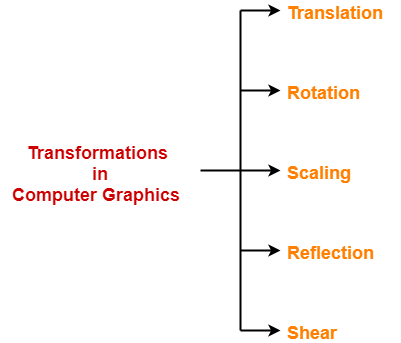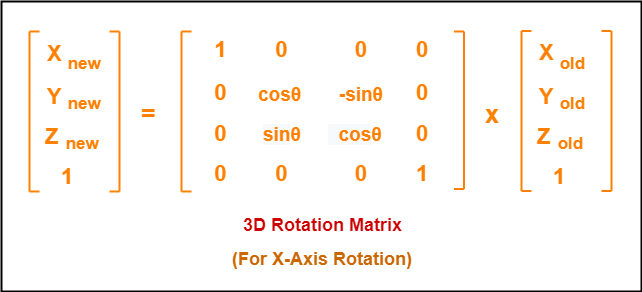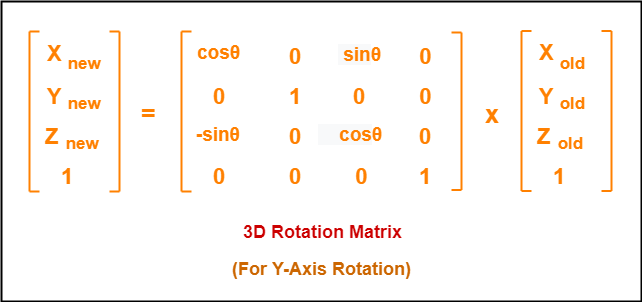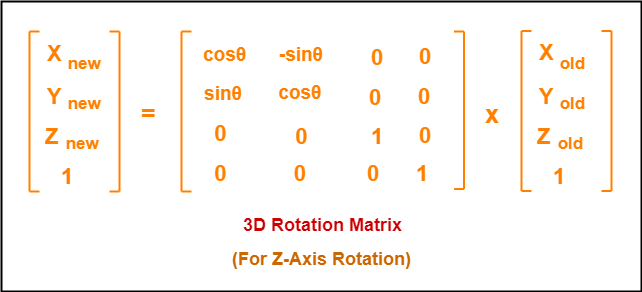# 3D Rotation in Computer Graphics | Definition | Examples

## 3D Transformations in Computer Graphics-

We have discussed-

• Transformation is a process of modifying and re-positioning the existing graphics.
• 3D Transformations take place in a three dimensional plane.

In computer graphics, various transformation techniques are-## 3D Rotation in Computer Graphics-

 In Computer graphics, 3D Rotation is a process of rotating an object with respect to an angle in a three dimensional plane.

Consider a point object O has to be rotated from one angle to another in a 3D plane.

Let-

• Initial coordinates of the object O = (Xold, Yold, Zold)
• Initial angle of the object O with respect to origin = Φ
• Rotation angle = θ
• New coordinates of the object O after rotation = (Xnew, Ynew, Znew)

In 3 dimensions, there are 3 possible types of rotation-

• X-axis Rotation
• Y-axis Rotation
• Z-axis Rotation

### For X-Axis Rotation-

This rotation is achieved by using the following rotation equations-

• Xnew = Xold
• Ynew = Yold x cosθ – Zold x sinθ
• Znew = Yold x sinθ + Zold x cosθ

In Matrix form, the above rotation equations may be represented as-### For Y-Axis Rotation-

This rotation is achieved by using the following rotation equations-

• Xnew = Zold x sinθ + Xold x cosθ
• Ynew = Yold
• Znew = Yold x cosθ – Xold x sinθ

In Matrix form, the above rotation equations may be represented as-### For Z-Axis Rotation-

This rotation is achieved by using the following rotation equations-

• Xnew = Xold x cosθ – Yold x sinθ
• Ynew = Xold x sinθ + Yold x cosθ
• Znew = Zold

In Matrix form, the above rotation equations may be represented as-## Problem-01:

Given a homogeneous point (1, 2, 3). Apply rotation 90 degree towards X, Y and Z axis and find out the new coordinate points.

## Solution-

Given-

• Old coordinates = (Xold, Yold, Zold) = (1, 2, 3)
• Rotation angle = θ = 90º

### For X-Axis Rotation-

Let the new coordinates after rotation = (Xnew, Ynew, Znew).

Applying the rotation equations, we have-

• Xnew = Xold = 1
• Ynew = Yold x cosθ – Zold x sinθ = 2 x cos90° – 3 x sin90° = 2 x 0 – 3 x 1 = -3
• Znew = Yold x sinθ + Zold x cosθ = 2 x sin90° + 3 x cos90° = 2 x 1 + 3 x 0 = 2

Thus, New coordinates after rotation = (1, -3, 2).

### For Y-Axis Rotation-

Let the new coordinates after rotation = (Xnew, Ynew, Znew).

Applying the rotation equations, we have-

• Xnew = Zold x sinθ + Xold x cosθ = 3 x sin90° + 1 x cos90° = 3 x 1 + 1 x 0 = 3
• Ynew = Yold = 2
• Znew = Yold x cosθ – Xold x sinθ = 2 x cos90° – 1 x sin90° = 2 x 0 – 1 x 1 = -1

Thus, New coordinates after rotation = (3, 2, -1).

### For Z-Axis Rotation-

Let the new coordinates after rotation = (Xnew, Ynew, Znew).

Applying the rotation equations, we have-

• Xnew = Xold x cosθ – Yold x sinθ = 1 x cos90° – 2 x sin90° = 1 x 0 – 2 x 1 = -2
• Ynew = Xold x sinθ + Yold x cosθ = 1 x sin90° + 2 x cos90° = 1 x 1 + 2 x 0 = 1
• Znew = Zold = 3

Thus, New coordinates after rotation = (-2, 1, 3).

To gain better understanding about 3D Rotation in Computer Graphics,

Watch this Video Lecture

Next Article- 3D Scaling in Computer Graphics

Get more notes and other study material of Computer Graphics.

Watch video lectures by visiting our YouTube channel LearnVidFun.

SummaryArticle Name
3D Rotation in Computer Graphics | Definition | Examples
Description
3D Rotation in Computer Graphics is a process of rotating an object with respect to an angle in 3D plane. Rotation in Computer Graphics Definition, Solved Examples and Problems.
Author
Publisher Name
Gate Vidyalay
Publisher Logo